# August 23rd Collectibles and Antiques

### Auction Details

Collectibles and Antiques
Choose Your Layout >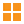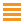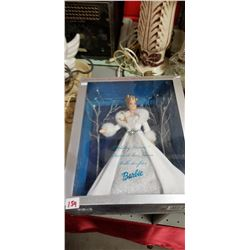154 - Shelf One
CDC Auctions
Shelf One
Bidding Has Concluded

Sold to f***r for (12.50 + 1.63) x 1 = 14.13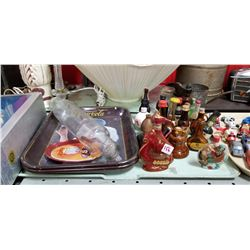156 - Shelf One
CDC Auctions
Shelf One
Bidding Has Concluded

Sold to f***r for (5.00 + 0.65) x 1 = 5.65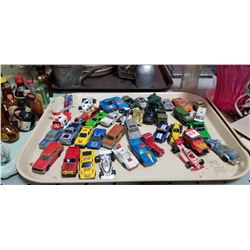157 - Shelf One
CDC Auctions
Shelf One
Bidding Has Concluded

Sold to f***r for (15.00 + 1.95) x 1 = 16.95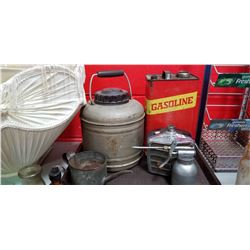158 - Shelf One
CDC Auctions
Shelf One
Bidding Has Concluded

Sold to f***r for (5.00 + 0.65) x 1 = 5.65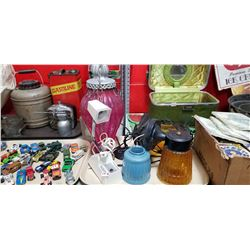159 - Shelf One
CDC Auctions
Shelf One
Bidding Has Concluded

Sold to f***r for (5.00 + 0.65) x 1 = 5.65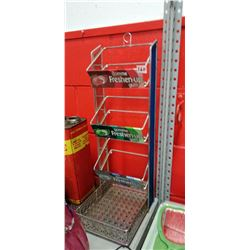160 - Shelf Two
CDC Auctions
Shelf Two
Bidding Has Concluded

Sold to f***r for (7.50 + 0.98) x 1 = 8.48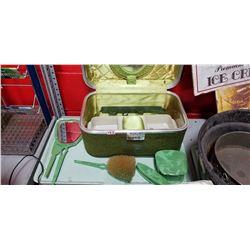161 - Shelf One
CDC Auctions
Shelf One
Bidding Has Concluded

Sold to b*******W for (20.00 + 2.60) x 1 = 22.60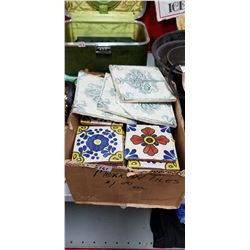162 - Shelf One
CDC Auctions
Shelf One
Bidding Has Concluded

Sold to f***r for (5.00 + 0.65) x 1 = 5.65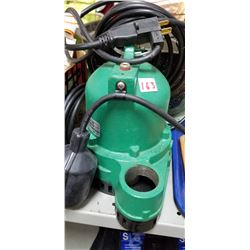163 - Shelf One
CDC Auctions
Shelf One
Bidding Has Concluded

Sold to f***r for (60.00 + 7.80) x 1 = 67.80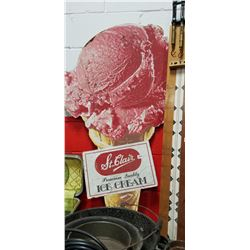165 - Shelf One
CDC Auctions
Shelf One
Bidding Has Concluded

Sold to f***r for (15.00 + 1.95) x 1 = 16.95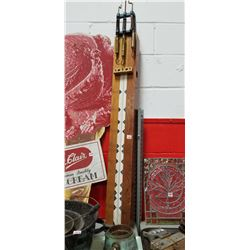166 - Shelf One
CDC Auctions
Shelf One
Bidding Has Concluded

Sold to f***r for (17.50 + 2.28) x 1 = 19.78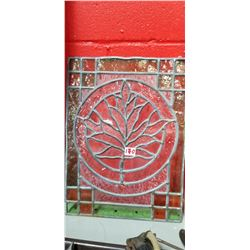170 - Shelf One
CDC Auctions
Shelf One
Bidding Has Concluded

Sold to f***r for (5.00 + 0.65) x 1 = 5.65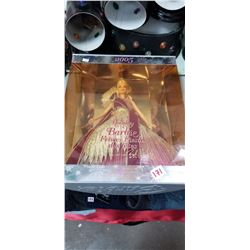171 - Shelf One
CDC Auctions
Shelf One
Bidding Has Concluded

Sold to f***r for (5.00 + 0.65) x 1 = 5.65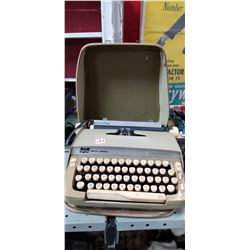173 - Shelf One
CDC Auctions
Shelf One
Bidding Has Concluded

Sold to f***r for (5.00 + 0.65) x 1 = 5.65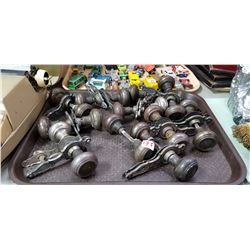175 - Shelf One
CDC Auctions
Shelf One
Bidding Has Concluded

Sold to b*******W for (10.00 + 1.30) x 1 = 11.30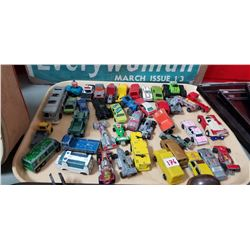176 - Shelf One
CDC Auctions
Shelf One
Bidding Has Concluded

Sold to f***r for (10.00 + 1.30) x 1 = 11.30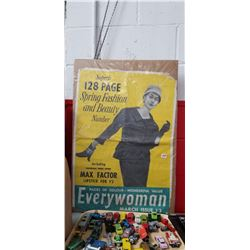177 - Shelf One
CDC Auctions
Shelf One
Bidding Has Concluded

Sold to f***r for (5.00 + 0.65) x 1 = 5.65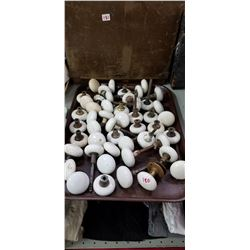180 - Shelf One
CDC Auctions
Shelf One
Bidding Has Concluded

Sold to b*******W for (12.50 + 1.63) x 1 = 14.13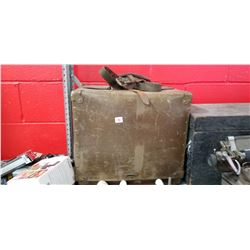181 - Shelf One
CDC Auctions
Shelf One
Bidding Has Concluded

Sold to f***r for (5.00 + 0.65) x 1 = 5.65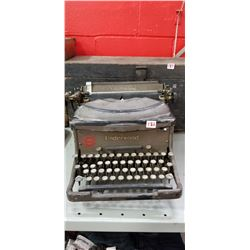182 - Shelf One
CDC Auctions
Shelf One
Bidding Has Concluded

Sold to f***r for (12.50 + 1.63) x 1 = 14.13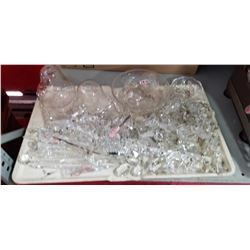184 - Shelf One
CDC Auctions
Shelf One
Bidding Has Concluded

Sold to f***r for (5.00 + 0.65) x 1 = 5.65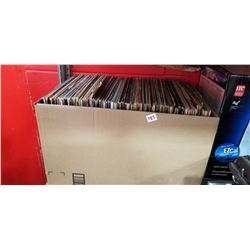185 - Shelf One
CDC Auctions
Shelf One
Bidding Has Concluded

Sold to f***r for (5.00 + 0.65) x 1 = 5.65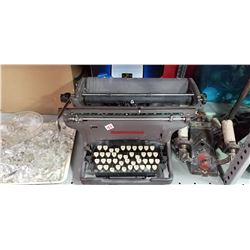186 - Shelf One
CDC Auctions
Shelf One
Bidding Has Concluded

Sold to f***r for (5.00 + 0.65) x 1 = 5.65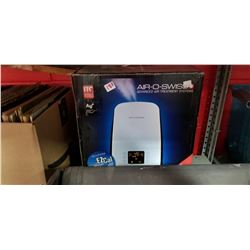187 - Shelf One
CDC Auctions
Shelf One
Bidding Has Concluded

Sold to f***r for (5.00 + 0.65) x 1 = 5.65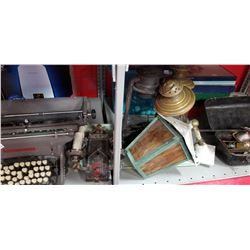188 - Shelf One
CDC Auctions
Shelf One
Bidding Has Concluded

Sold to f***r for (7.50 + 0.98) x 1 = 8.48189 - Shelf One
CDC Auctions
Shelf One
Bidding Has Concluded

Sold to f***r for (5.00 + 0.65) x 1 = 5.65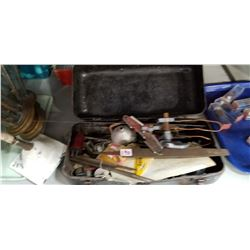190 - Shelf One
CDC Auctions
Shelf One
Bidding Has Concluded

Sold to f***r for (5.00 + 0.65) x 1 = 5.65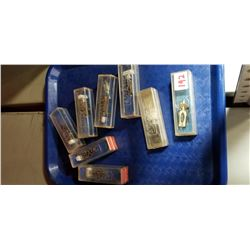192 - Shelf One
CDC Auctions
Shelf One
Bidding Has Concluded

Sold to f***r for (20.00 + 2.60) x 1 = 22.60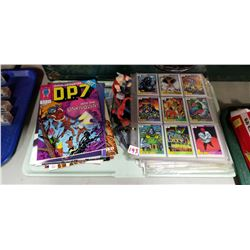193 - Shelf One
CDC Auctions
Shelf One
Bidding Has Concluded

Sold to f***r for (10.00 + 1.30) x 1 = 11.30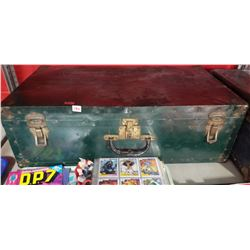194 - Shelf One
CDC Auctions
Shelf One
Bidding Has Concluded

Sold to f***r for (5.00 + 0.65) x 1 = 5.65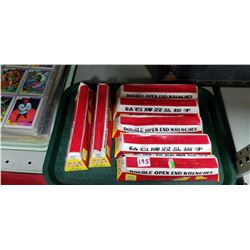195 - Shelf One
CDC Auctions
Shelf One
Bidding Has Concluded

Sold to f***r for (5.00 + 0.65) x 1 = 5.65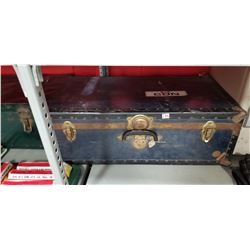196 - Shelf Two
CDC Auctions
Shelf Two
Bidding Has Concluded

Sold to f***r for (5.00 + 0.65) x 1 = 5.65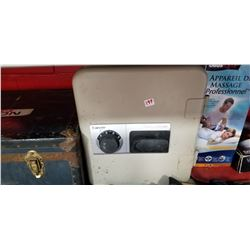197 - Shelf One
CDC Auctions
Shelf One
Bidding Has Concluded

Sold to f***r for (12.50 + 1.63) x 1 = 14.13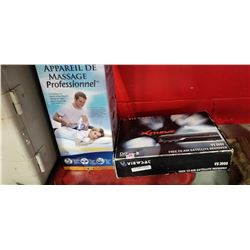198 - Shelf One
CDC Auctions
Shelf One
Bidding Has Concluded

Sold to f***r for (5.00 + 0.65) x 1 = 5.65